﻿ Modeling of the Garification Process of Fermented Cassava Mash

Like us on:

# Modeling of the Garification Process of Fermented Cassava Mash

Sobowale SS1*, Awonorin SO2, Shittu TA2, Ajisegiri EA3, Adebo OA4 and Olatidoye OP5
1Department of Food Technology, Moshood Abiola Polytechnic, Nigeria
2Department of Food Science and Technology, Federal University of Agriculture, Nigeria
3Department of Agricultural Engineering, Federal University of Agriculture, Nigeria
4Department of Biotechnology and Food Technology, Faculty of Science, University of Johannesburg, South Africa
5Department of Food Technology, Yaba College of Technology, Nigeria
*Corresponding Author: Sobowale SS, Department of Food Technology, Moshood Abiola Polytechnic, Nigeria, Tel: 2348033791755, Email: [email protected]

Received Date: Oct 10, 2017 / Accepted Date: Oct 25, 2017 / Published Date: Oct 30, 2017

### Abstract

This study was carried out to analyze the garification process using Artificial Neural Network (ANN) based model of steady state simultaneous heat and mass transfer. Convective heat and mass transfer coefficients were obtained during garification process of fermented mash from cassava ages of different maturity. Empirical equations developed for heat, (hc) and mass, (hm) transfer coefficients [hc=0.017t2-0.388t+3.039, hm=0.042t2-0.914t+5.481]; with (R2>0.9) were best described by polynomial relationships. The optimum ANN model that produced convective heat and mass transfer coefficients for the garification process consisted of two hidden layers and twenty-five neurons in each hidden layer, with mean square error, mean absolute error, sum square error and R2 of 0.000015, 0.0030, 0.0082% and 0.995, respectively. The developed ANN model can be useful in the determination of heat and mass transfer rate for garification process and wide range of physical conditions. These results are equally important considerations for obtaining quality gari for commercial production.

Keywords: Gari; Garification; Artificial neural network; Convective heat; Mass transfer coefficient

#### Introduction

Cassava (Manihot esculenta Crantz) is a staple food for over one billion people in the developing world . In Nigeria, it appears to be the major staple food that matches population growth [2,3]. They are highly perishable and cannot be kept after harvest, hence it can either be consumed immediately or processed into various product [2,3]. The main form in which cassava is eaten in West Africa is in a roasted granular form known as gari, a fermented roasted granule prepared from peeled, grated and fermented cassava root through a series of processing steps [3-5]. The quality and yield of gari during roasting (garification) is affected by cassava root age and length of cassava mash fermentation. The garification process is an arduous and intricate operation which needs good understanding of the factors that affect the yield and quality of the gari . In recent years, the inefficacy of the process coupled with exposure of the operator to heat and smoke have been the major factors reducing the efficiency of the gari roasting in the traditional methods. The traditional gari processing methods not only gives relatively good quality, but it is highly labour-intensive, unhygienic and productivity is often too low to justify the investment of labour, time consuming and leads itself to health hazard for the operator . Engineering interventions to improve the industrial process is often by way of reducing drudgery and maximizing the yield of gari and improve quality through the mechanization of the unit operations. Considerations for heat and mass transfer are also necessary to improve the working conditions of gari processing for sustainable economic development in Nigeria. The study of  has shown that the heat and mass transfer operations play a prominent role during reconstitution of gari into thick paste, and are among the most important physical phenomena occurring during gari processing. As a consequence, several important variables such as temperature and moisture concentration within the structure of food depend on time as well as position inside the food system  highlighted that gari yield and quality are highly influenced by cassava variety, maturity age, fermentation and garification temperature, convective heat and mass transfer coefficients. Mathematical modeling and computer-based numerical analyses such as Partial Differential Equation (PDE), Response Surface Methodology (RSM) have been extensively used for time and cost reduction in experimentation, design, optimization and validation of food processing operations . Previous studies of Baik [11,12] and Singh and Pandey , have shown that ANN model is an advanced modified numerical analysis method, which is capable of learning from examples through iteration and could be useful in predicting simultaneous heat and mass transfer data. ANN model was applied in this study to analyze the garification process and to develop an ANN based model of steady state simultaneous heat and mass transfer for the garification process of fermented cassava mash.

#### Materials And Methods

Mathematical modeling

In order to successfully model the garification process of fermented mash from cassava ages of maturity, a few assumptions were made. The assumptions aided the derivation of the mathematical model which was compared with the experimental data in the previous studies [3,8,14] in an attempt to validate or otherwise reject the assumptions. The assumptions were as follows; (i) The initial moisture and temperature distribution in the sample was uniform, (ii) Moisture movement and heat distribution was by diffusion and conduction, respectively, (iii) The effective moisture and thermal diffusivities was determined experimentally taken into account other possible mechanisms of the garification process, (iv) Temperature and moisture gradients within individual particles are negligible, (v) The particles of the fermented cassava mash are assumed spherical in shape and of uniform moisture content. Based on the earlier described assumptions, the mathematical numerical model characterizing one–dimensional heat and mass transfer data during garification process of fermented mash from cassava ages of maturity is presented as follows:

Heat transfer governing equation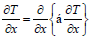(1)

Mass transfer governing equation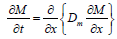(2)

where, T=Temperature (°C), t=Time (s), M=Moisture content in mass fraction (dry basis), α=Thermal diffusivity, m2/s, Dm=Moisture diffusivity, m2/s.

Determination of convective heat and mass transfer coefficients

The convective heat and mass transfer coefficients was estimated from the corresponding thermo-physical data and estimated dimensionless numbers.

Heat transfer coefficient (hc)

NNu=hcd/ks=f (NRe)m.(NPr)n (For forced convection) (3)

NNu=hcd/ks=α [(NGr).(NPr)]C (For natural convection) (4)

∴ hc=NNU.Ks/d (5)

Mass transfer coefficient (hm)

NSh=hmd/Dm=f (NRe)m.(NSc)n (For forced convection) (6)

NSh=hmd/Dm=α [(NGr).(NSc)]C (For natural convection) (7)

∴ hm=Nsh.Dm/d (8)

The values of f, m, and n are the function of (NRe.NPr) and (NRe.NSc), while α and c is the function of (NGr.NPr) and (NGr.NSc) dimensionless parameters. NNu=Nusselt number, hcd/ks;NRe=Reynolds number, ρdV/μ; NPr=Prandtl number, Cpμ/K; NGr=Grashof number=d3ρ2gβΔT/ μ2; NSh=Sherwood number, hm d/Dm; NSc=Schmidt number, μ/ dρ;ks=Thermal conductivity of the sample; d=Mean diameter of the particle size of sample; Dm=Diffusion coefficient (Mass diffusivity).

Initial conditions

M (x, 0)=M°

T(x, 0)=T°

Boundary conditions

For heat transfer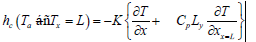(9)

For mass transfer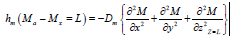(10)

where, hc=Convective heat transfer coefficient, hm=Convective mass transfer coefficient, L=latent heat of vapourization, x=Dimensional co-ordinate in x direction, y=Dimensional co-ordinate in y direction, z=Dimensional co-ordinate in z direction.

Finite difference equations: A one-dimension slab finite difference framework was developed to convert partial differential equations into ordinary differential equations as shown in Figure 1.

Figure 1: Topological architectural structure of Artificial Neural Network model for the garification process of fermented mash from cassava ages of maturity.

Temperature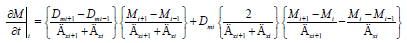(11)

Moisture: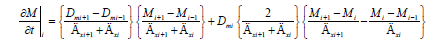(12)

To solve the heat and mass transfer algebraic equation simultaneously, the Artificial Neural Network (ANN) model, an iterative numerical method was developed using the method described by Caudill  to analyze the heat and mass transfer data during garification process of fermented mash from cassava ages of maturity. A MATLAB commercial software package [Version 7.10.0 (R 2010a) Neural Works Professional II/Plus (Neural Ware, Pittsburg, PA)] was used for the development of the neural network model which involves the generation of (or compilation of available) data required for training/ testing, the training/testing of the ANN model, evaluation of the ANN configuration leading to the selection of an optimal configuration and validation of the optimal ANN model with data set not used in the training [16,17]. The procedure used for the development of our ANN model is outlined below.

Data generation

Many researchers have measured thermo-physical properties of foods such as thermal conductivity, thermal diffusivity, moisture diffusivity by various techniques. Some comprehensive reviews on the data and prediction models of thermo-physical properties have been published by several researchers [18,19]. Convective heat and mass transfer coefficients data have been presented and modeled during the garification process. The convective heat and mass transfer coefficients data for the garification process were obtained from the experimental work [3,8,14] where fermentation period, garification temperature and time and cassava age of maturity, were the information available. The datasets were organized in four columns representing fermentation period, garification temperature, garification time and cassava age of maturity, with the parameters P, RT, Rt and V, respectively as independent (input) variables, while convective heat (hc) and mass transfer (hm) coefficients were the dependent (output) variables. ANN models were developed and trained using these datasets. In each instance, the complete dataset was used to assess the prediction capability of a given model.

Training of ANN

Several ANN models were trained using the experimental data set. The feed forward network structure with input, hidden layer(s) was used in this study as shown in Figure 2. The input layer consisted of four (4) neurons which corresponded to fermentation period, garification temperature and time and cassava age of maturity, while the output layers had two neurons representing the convective heat and mass transfer coefficients. The number of hidden layers and neurons within each hidden layer can be varied based on the complexity of the problem and data set. In this study, the number of hidden layers was varied from 1 to 2. The neurons within each of these layers varied from 5 to 40 with increments of five. This resulted in a total of 16 networks. The optimal configuration was based upon minimizing the difference between the neural networks predicted values and the actual outputs. The data sets of 441 cases from the experimental method were divided in two sets. The first set consisted of 353 (80%) cases for training/testing and 88 (20%) cases for validation, chosen randomly from the set of 441 cases. A back-propagation network algorithm was utilized in model training. A log sigmoid transfer function was used for input layers while pure-line transfer function was used for output layers. The error minimization process was achieved using gradient descent rule [20-22]. To avoid the potential problem of over-training or memorization while employing feed forward algorithm, the option of saving the best configuration was selected where the network with the best result is saved during the selected long number of training cycles of 100,000.

Figure 2: Finite difference grid of a one - dimensional rectangular region. The nodes which are involved in the computation of the temperature at position (i, j) are indicated by dots. where, P=Fermentation period, RT=Garification temperature, Rt=Garification time, v=Cassava age of maturity.

Selection of optimal configuration

The optimal configuration was selected from 16 ANN configurations based on minimizing three error measures used to assess the predictive performance of each configuration. The performances of the various ANN configurations were compared using mean square error (MSE), mean absolute error (MAE) and sum square error (SSE). The coefficient of determination R2, of the linear regression line between the predicted values from the neural network model and the desired output was also used as a measure of performance. The three different indicators of the model fit were defined in these equations as follows: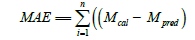(13)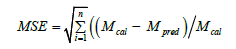(14)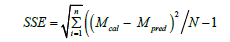(15)

where,Mcal=Desired/calculated output by experiment, Mpred=Predicted value by the model and N=No of data points.

#### Results And Discussions

Data needed for development for the ANN model were obtained from our recent experimental work analysis of heat and mass transfer of garification process of fermented mash from cassava ages of maturity as shown in Figures 3 and 4. Empirical equations developed for heat, (hc) and mass, (hm) transfer coefficients [hc=0.017t2-0.388t+3.039, hm=0.042t2-0.914t+5.481]; with (R2>0.9) were best described by polynomial relationships. Once a given ANN configuration was trained using the input data, its performance was evaluated with the same data set. The analysis was repeated in triplicate. The ANN configuration (out of 16) that minimized the three error measures to determine its performance, and optimized R2, was selected as the optimum. The optimum ANN configuration included two hidden layers with twentyfive neurons in each layer. The MSE, MAE and SSE for this optimal configuration with different neural networks were 0.000015, 0.0030 and 0.0082%, respectively, and had R2 of 0.995. The simplest ANN model with one hidden layer and five neurons predicted convective heat and mass transfer coefficients with 0.00046% MSE, 0.0105% MAE and 0.22% SSE and had R2 of 0.972, as shown in Table 1. The test error which determines the amount of weight changes during series of iterations to bring the predicted value within the acceptable range of the experimental values were adjusted within the hidden layers and neurons on trial basis [21,22]. The topology which gave the minimum error in the minimum number of iterations during the training of the ANN was selected. The preliminary trial of the test error indicated that higher learning rates produced poorly developed models. These observations were similar with the published work. However, the magnitude of the errors was about the same (~10%) as the variation was significant as compare to the original experimental data. The prediction accuracy did not increase with increasing network parameters. The optimal network with two hidden layers and twenty-five neurons in each hidden layer were considered as a good predictor and this model is also recommended to users. have developed ANN based model for heat transfer during reconstitution of gari granules into a paste. It had an SSE of 0.0085% and R2>0.9. The ANN model developed in this present study could capture the variation of heat and mass transfer data during garification process of fermented mash. In addition, the developed ANN model could be useful in the determination of heat and mass transfer rate for the garification process and wide range of physical conditions.

Figure 3: Surface plot of effect of heat transfer coefficient (W/m2 °C) of gari samples as influenced by cassava processing variables.

Figure 4: Surface plot of effect of mass transfer coefficient (m/s) of gari sample as influenced by cassava processing variables.

Hidden layer Neuron layer in each hidden layer MSE (%) MAE (%) SSE (%) R2
1 5 0.00046 0.0105 0.22 0.972*
1 10 0.00170 0.024 0.89 0.882
1 15 0.00099 0.0177 0.52 0.905
1 20 0.00078 0.0140 0.40 0.899
1 25 0.00068 0.0123 0.35 0.896
1 30 0.0120 0.052 0.38 0.197
1 35 0.00140 0.021 0.70 0.891
1 40 0.00042 0.009 0.23 0.864
2 5 0.00240 0.031 127 0.885
2 10 0.000152 0.0086 0.08 0.940
2 15 0.000044 0.0049 0.023 0.910
2 20 0.000037 0.0043 0.019 0.897
2 25 0.000015 0.0030 0.0082 0.995*
2 30 0.000053 0.0036 0.0093 0.867
2 35 0.00760 0.086 0.06 0.935
2 40 0.00452 0.0096 0.047 0.863

Table 1: Error Parameter in the prediction of convective heat and mass transfer coefficients with different neural network configurations.

#### Conclusions

An ANN model was developed for the garification process of fermented mash under a wide range of cassava processing variables. The optimum ANN model consisted of two hidden layers and twentyfive neurons in each hidden layer, with mean square error (MSE), means absolute error (MAE) and sum square error (SSE) of 0.000016%, 0.0029% and 0.0085%, respectively and had R2 of 0.992. This developed model is recommended and could be useful to estimate convective heat and mass transfer data for garification (roasting) process. These are important considerations to obtain an appreciable yield and quality gari for commercial production.

#### Acknowledgements

The main author (Sobowale SS) wishes to acknowledge the financial support received from the Tertiary Education Trust Fund (TETFUND) of the Nigerian Government under the management of Moshood Abiola Polytechnic, Abeokuta, Nigeria.

#### References

Citation: Sobowale SS, Awonorin SO, Shittu TA, Ajisegiri ES, Adebo OA, et al. (2017) Modeling of the Garification Process of Fermented Cassava Mash. J Bioprocess Biotech 7: 311. DOI: 10.4172/2155-9821.1000311

Copyright: © 2017 Sobowale SS, et al. This is an open-access article distributed under the terms of the Creative Commons Attribution License, which permits unrestricted use, distribution, and reproduction in any medium, provided the original author and source are credited.

Select your language of interest to view the total content in your interested language

##### Recommended Journals
Viewmore
###### Article Usage
• Total views: 1777
• [From(publication date): 0-2017 - Dec 08, 2019]
• Breakdown by view type
• HTML page views: 1670Can't read the image? click here to refresh